Related Articles

• Difficulty Level : Hard
• Last Updated : 28 Sep, 2020

Classification is a typical supervised learning task. We use it in those cases where we have to predict a categorical type, that is if a particular example belongs to a category or not (unlike regression, which is used to predict continuous values). For example, sentiment analysis, classify an email as spam or not, predicting if a person buys an SUV or not provided a training set containing salary, and buying an SUV.

Types of Classification Models:

Attention reader! Don’t stop learning now. Get hold of all the important Machine Learning Concepts with the Machine Learning Foundation Course at a student-friendly price and become industry ready.

• Logistic Regression is a linear classification model ( and hence, the prediction boundary is linear ), which is used to model binary dependent variables. It is used to predict the probability (p) that an event occurs. If p >= 0.5, the output is 1 else 0. The sigmoid function maps the probability value to the discrete classes (0 and 1). For example, say our logistic regression model is trained on the dataset containing a person’s salary and whether he buys an SUV or not. Now, given the person’s salary, our model predicts whether or not the person buys an SUV. Few of the assumptions of logistic regression are – there is no high inter-correlation among the predictors, there is a linear relationship between the sigmoid of the outcome and the predictor variables.
• K – Nearest Neighbours is a non – linear classifier (and hence, the prediction boundary is non-linear) that predicts which class a new test data point belongs to by identifying its k nearest neighbors’ class. We select these k nearest neighbors based on Euclidean distance. Among these k neighbours, the number of data points in each category is counted, and the new data point is assigned to that category where we got the most neighbours in.
• Support Vector Machine (SVM) is used as a linear or non-linear classifier based on the kernel used. If we use a linear kernel,  then the classifier and hence the prediction boundary are linear. Here, to separate two classes, we need to draw a line. The line is such that there is a maximum margin. This line is drawn equidistant from both the sets. We draw two more lines on either side, and these are known as support vectors. SVMs learn from the support vectors, unlike other machine learning models that learn from the correct and incorrect data. For example, suppose we have two classes – apples and oranges. In that case, SVM learns those examples which are rightmost in apples (an apple resembling an orange) and leftmost in oranges (an orange resembling an apple); that is, they look at the extreme cases. Therefore, they perform better most of the time.
• Kernel SVM is particularly useful when the data is not linearly separable. Therefore, we take our non – linearly separable dataset, map it to a higher dimension, get a linearly separable dataset, invoke SVM classifier, build a decision boundary for the data, and then project it back into original dimensions. This mapping can be computationally expensive, and hence we use the Kernel Trick, which gives similar results. The kernels available are – The Gaussian RBF Kernel, Sigmoid Kernel, Polynomial Kernel (default value of kernel is ‘RBF’). This is also known as the non – linear SVM.
• Naive Bayes Classifier works on the basis of Bayes’ Theorem. The fundamental assumptions made are that all the features are independent of one another and contribute equally to the outcome; all are of equal importance. But these assumptions are not always valid in real life (disadvantage of Naive Bayes). It is a probabilistic classifier model whose crux is the Bayes’ theorem.
• Decision Tree Classification is the most powerful classifier. A Decision tree is a flowchart like a tree structure, where each internal node denotes a test on an attribute (a condition), each branch represents an outcome of the test (True or False), and each leaf node (terminal node) holds a class label. Based on this tree, splits are made to differentiate classes in the original dataset given. The classifier predicts which of the classes a new data point belongs to based on the decision tree. The prediction boundaries are horizontal and vertical lines. Below is the data distribution and its corresponding decision tree.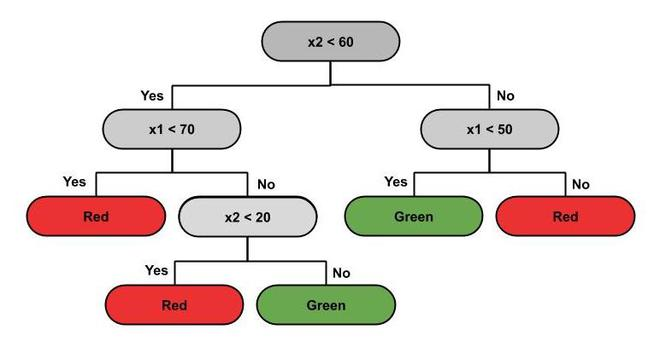Decision Tree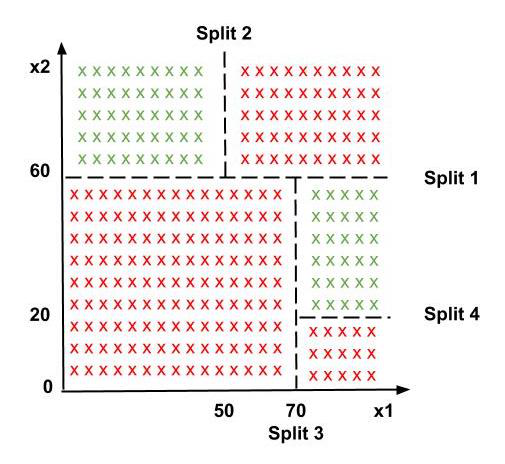Splitting the dataset using Decision Tree

• Random Forest Classification is an example of Ensemble learning, where multiple machine learning algorithms are put together to create one bigger and better performance ML algorithm. We randomly pick  ‘k’ data points from the training set, build a decision tree associated with these k points. Then, we choose the number of trees ‘n’ we want to build and repeat. For a new data point, we take the predictions of each of the ‘n’ decision trees and and assign it to the majority vote category.

How do we choose the right Classification Model for a given problem?

The accuracy of classification models is measured in terms of the number of false positives and negatives.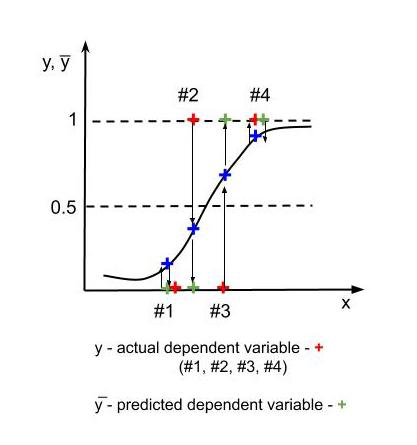False positives and False negatives

In the above figure, for 1, 4 – y =  y̅ ( actual value = predicted value). The error at 3 is False positive or type-1 error (we predicted a positive outcome, but it was false – we predicted an effect that did not occur). The error at 2 is False negative or type-2 error (we predicted an outcome false, which in reality happens – This is something like predicting that a cancer patient does not have cancer, which is very dangerous for the patient’s health. We use a Confusion Matrix to represent the number of false positives, false negatives, and correctly predicted outcomes.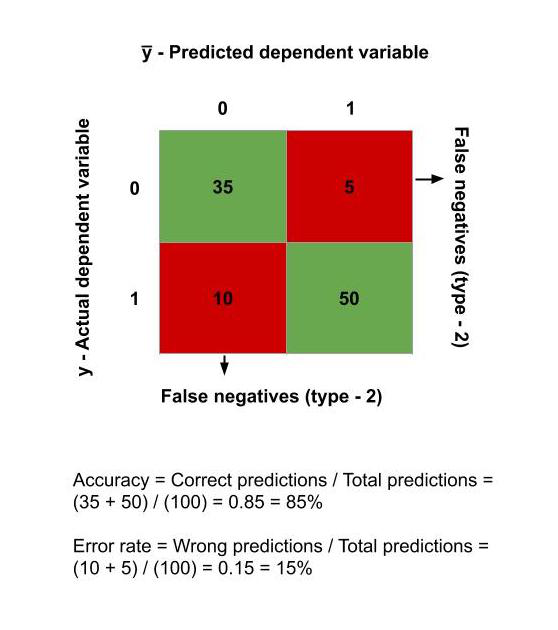Calculating Accuracy from Confusion Matrix

Suppose that initially, the model correctly predicts 9700 observations as true, 100 observations as false, 150 are type-1 errors (False positives) and the rest 50 are type-2 errors (False negatives). Hence, the accuracy rate = (9800/10000)*100 = 98%.

Now, let us stop the model from making predictions and say that our prediction y̅ = 0 always. In this case, the number of false positives reduces to 0 and adds to correctly predicted true observations, whereas previously correctly predicted false observations reduces to 0. It adds to the false negatives. Therefore, now we have – 9850 observations are correctly predicted as true, 150 observations are false negatives. Hence, the accuracy rate = (9850/10000)*100 = 98.5%, which is more than the previous model! But actually, our model is not trained at all. It is predicting 0 always. This is known as Accuracy Paradox.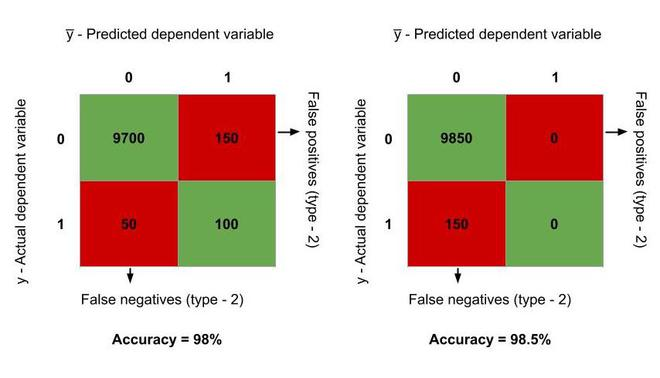Therefore, we need more accurate methods than the accuracy rate to analyse our model. We use the CAP curve for this purpose. The Accuracy ratio for the model is calculated using the CAP Curve Analysis. The accuracy ratio is given as the ratio of the area enclosed between the model CAP and the random CAP (aR) to the area enclosed between the Perfect CAP and  the random CAP (aP). The closer the accuracy ratio is to 1, the better the model is. A good model has its CAP curve between the perfect CAP and the random CAP.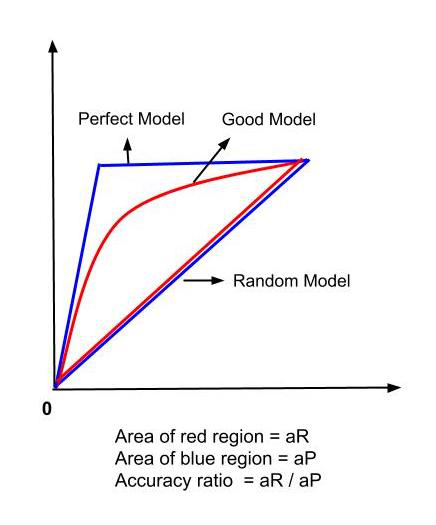CAP Curve Analysis

By considering the type of relation between the dependent and independent variable (linear or non-linear), the pros and cons of choosing a particular classification model for the problem, and the accuracy of the model through the methods mentioned above, we choose the classification problem that is the most suitable to the problem to be solved.

My Personal Notes arrow_drop_up